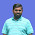Menu

# Kerala PSC LDC Exam 2013 Coaching

PART- A

### General Knowledge Questions and answers related to Electricity for preparing Kerala PSC Lower Division Clerk [LDC] Examination 2013.

1. The law of electrolysis was formulated by?
Answer: Michael Faraday

2. Father of Electricity?
Answer: Michael Faraday

3. The ordered flow of charges in a conductor is called?
Answer: Electric current

4. The unit of current?
Answer: Ampere

5. The substance that allows electric charges to flow through it?
Answer: Conductor

6. The best conductor of Electricity?
Answer: Silver

7. The substance that does not allow current to flow through it?
Answer: Insulators

8. The unit of electric charge?
Answer: Coulomb

9. A device that maintains a constant potential difference across its terminals for a long time?
Answer: Cell

10. The fuse is a piece of wire made of?
Answer: Bismuth alloy

11. The instrument used to measure electric current in a circuit is called?
Answer: Ammeter

12. Unit of electric potential?
Answer: Volt

13. Commercial unit of electricity?
Answer: KWH (Kilowatt Hour)

14. An instrument used to measure the electrical energy consumed in Kwh?
Answer: Watt-hour meter

15. The potential difference measured in volt is called?
Answer: Voltage

16. The EMF of a dry cell is:
Answer: 1.5 volt

17. The source of EMF containing two or more cells is called?
Answer: Battery

18. The electric current which changes its direction at regular intervals of time?
Answer: Alternating Current (AC)

19. Voltage of AC in our household circuit?
Answer: 230 V

20. Electric current flowing always in the same direction?
Answer: Direct Current (DC)

21. The reaction in torch cell is?
Answer: Electrochemical

22. ‘Hydro power’ is the term used for electricity produced through?
Answer: Water

#### Post a Comment

1.Does the LDC exam notification arrive?Or is it already published?

1.Kerala PSC LDC Exam 2013 Notification expect to be on July 2013.

2.pls sendsomemathematical questions and answers

3.pls send mathematical questions and answers

Top